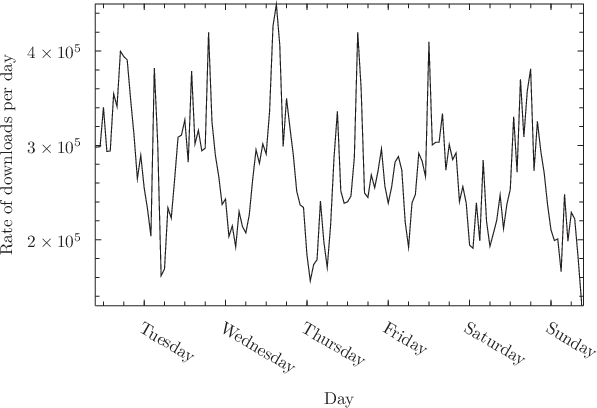## 4.11.2 Time intervals

The time interval between two date objects can be found by subtracting one from the other. The following example calculates the time interval between Albert Einstein’s birth and death. The result is returned as a numerical object with physical dimensions of time:

pyxplot> myDate1 = time.fromCalendar(1879,3,14,0,0,0)
pyxplot> myDate2 = time.fromCalendar(1955,4,18,0,0,0)
pyxplot> print myDate2 - myDate1
2401315200 s
pyxplot> print (myDate2 - myDate1) / unit(year)
76.094714

The function time.interval(t1,t2) has the same effect. The next example calculate the time elapsed between the traditional date for the foundation of Rome by Romulus and Remus in 753 BC and that of the deposition of the last Emperor of the Western Empire in AD 476:

pyxplot> x = time.fromCalendar(-752,4,21,12,0,0)
pyxplot> y = time.fromCalendar( 476,9, 4,12,0,0)
pyxplot> print y-x
3.8764483e+10 s
pyxplot> print time.interval(y,x)
3.8764483e+10 s
pyxplot> print (y-x)/unit(year)
1228.3986

The function time.intervalStr() is similar, but returns a textual representation of the time interval. It takes an optional third parameter which specifies the textual format in which the time interval should be represented. If no format is supplied, then the following verbose format is used:
"%Y years %d days %h hours %m minutes and %s seconds"
Table 4.3 lists the tokens which are substituted for various parts of the time interval. The following examples demonstrate the use of the function:

 Token Substitution value %% A literal % sign. %d The number of days elapsed, modulo 365. %D The number of days elapsed. %h The number of hours elapsed, modulo 24. %H The number of hours elapsed. %m The number of minutes elapsed, modulo 60. %M The number of minutes elapsed. %s The number of seconds elapsed, modulo 60. %S The number of seconds elapsed. %Y The number of years elapsed.
Table 4.3: Tokens which are substituted for various components of the time interval by the time_diff_string function.

pyxplot> x = time.fromCalendar(-752,4,21,12,0,0)
pyxplot> y = time.fromCalendar( 476,9, 4,12,0,0)
pyxplot> print time.intervalStr(y,x)
pyxplot> print time.intervalStr(y,x,"\$%Ymathrm{y}%dmathrm{d}\$")
\$-1229mathrm{y}-78mathrm{d}\$

Example: A plot of the rate of downloads from an Apache webserver

In this example, we use Pyxplot’s facilities for handling dates and times to produce a plot of the rate of downloads from an Apache webserver based upon the download log which it stores in the file /var/log/apache2/access.log. This file contain a line of the following form for each page or file requested from the webserver:
127.0.0.1 - - [14/Jun/2012:16:43:18 +0100] "GET / HTTP/1.1" 200 484 "-" "Mozilla/5.0 (X11; Linux x86_64) AppleWebKit/535.19 (KHTML, like Gecko) Ubuntu/12.04 Chromium/18.0.1025.151 Chrome/18.0.1025.151 Safari/535.19"
However, Pyxplot’s default input filter for .log files (see Section 5.1) manipulates the dates in strings such as these into the form
127.0.0.1 - -  [ 14  6  2012 16 43 18 +0100 ]  "GET   HTTP 1.1" 200 484 "-" "Mozilla/5.0 (X11; Linux x86_64) AppleWebKit/535.19 (KHTML, like Gecko) Ubuntu/12.04 Chromium/18.0.1025.151 Chrome/18.0.1025.151 Safari/535.19"
such that the day, month, year, hour, minute and second components of the date are contained in the 5th to 10th white-space-separated columns respectively. In the script below, the time.fromCalendar() function and toUnix() method are then used to convert these components into Unix times. The histogram command (see Section 5.9) is used to sort each of the web accesses recorded in the Apache log file into hour-sized bins. Because this may be a time-consuming process for large log files on busy servers, we use the tabulate command (see Section 5.5) to store the data into a temporary data fileon disk before deciding how to plot it:
set output ’apache.dat’
histogram f() ’/var/log/apache2/access.log’using time.fromCalendar(\$7,\$6,\$5,\$8,\$9,\$10).toUnix()binwidth 1/24
tabulate f(x) with format "%16f %16f"
Having stored our histogram in the file apache.dat, we now plot the resulting histogram, labelling the horizontal axis with the days of the week. The commands used to achieve this will be introduced in Chapter 8. The major axis ticks along the horizontal axis are placed at daily intervals, and minor axis ticks are placed along the axis every quarter day, i.e. every six hours.
set width 10
set xlabel ’Day’